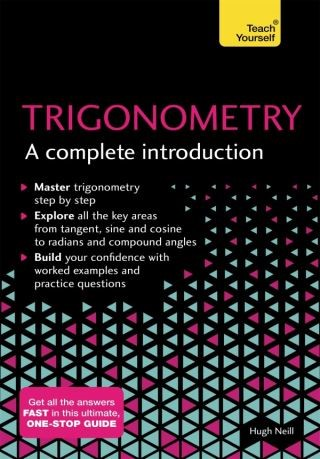## Trigonometry: A Complete Introduction: Teach Yourself• Description
• Author
• Info
• Reviews

### Description

Trigonometry: A Complete Introduction is the most comprehensive yet easy-to-use introduction to Trigonometry. Written by a leading expert, this book will help you if you are studying for an important exam or essay, or if you simply want to improve your knowledge.

The book covers all areas of trigonometry including the theory and equations of tangent, sine and cosine, using trigonometry in three dimensions and for angles of any magnitude, and applications of trigonometry including radians, ratio, compound angles and circles related to triangles. Everything you will need is here in this one book. Each chapter includes not only an explanation of the knowledge and skills you need, but also worked examples and test questions.